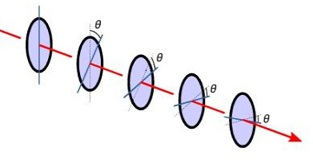# A beam of unpolarized light shines on a stack of five ideal polarizers, set up so that the...

## Question:

A beam of unpolarized light shines on a stack of five ideal polarizers, set up so that the angles between the polarization axes of pairs of adjacent polarizers are all equal. The intensity of the transmitted beam is reduced from the intensity of the initial beam by a factor of {eq}\phi \ = 0.145 {/eq}. Find the angle {eq}\theta {/eq} ( in degrees) between the axes of each pair of adjacent polarizers## : Polarization of light:

Polarization is a phenomenon in which an unpolarized light is converted to a plane polarized light by passing it through a polarizer, which therefore reduce the intensity to half. On further passing the polarized light through polarizer, intensity gets reduced, which is given by Malus law.

Become a Study.com member to unlock this answer! Create your account

when unpolarized light wave of intensity I passes through first polarizer, its intensity becomes half.

Therefore, intensity of light after passing...